# Results and code

The following shows the steps, as well as the Python code, required to reproduce the results in the paper. This has been ellaborated using the IPython notebook. You can read through the page below, download the source file or a pdf version.

## Access and software requirements¶

To reproduce the code below you will need to have installed the following requirements:

In :
```%matplotlib inline
import os
import pandas as pd
import employment_centers_tools as tools
```

## Data¶

Employment data for 1990 and 2000 comes from the “Census 2000 Special Tabulation Product 64” (`stp64`), which offers tract- to-tract commuting flows for the whole country. We consider only tracts within boundaries of the MSAs studied and sum over inflows to obtain employment numbers at the tract level. For 2010, there is no `stp64` so we use the Census Transportation Planning Products (CTPP) 5-year small area data, which is based on ACS 2006-2010 Census Data and provides employment counts at the tract level for 2010.

Since it is not clear to the authors whether it is legal to redistribute original Census data (particularly when the `stp64` is not available as a free download), the raw dataset used for this paper is not included. However, in order to illustrate how the process was carried out, below we show the structure of the data and how that is entered into the code to identify employment centers. This should allow users who have accessed the original data from the Census to replicate our results. We do provide the output of the center identification in the form of shapefiles (one per MSA per year). These can be found in the repository where this notebook and other code is hosted.

We store employment data in `csv` files, using a separate one for each MSA for each year. The raw format of these files is as follows, where the top of the file for MSA 10180 in 1990 is shown:

In :
```!head /Users/dani/AAA/LargeData/T-CentersData/attributes/3-empDen/empDen1990/10180.csv
```
```gisjoin2,emp,area,dens,densEB
48044100102,2855.0,45088315.8862,6.33201738385e-05,6.32174061116e-05
48044100105,357.0,16138974.527,2.212036455e-05,2.20337682175e-05
48044100104,272.0,18017057.8625,1.5096804488e-05,1.56769988243e-05
48044100107,565.0,18156353.5203,3.11185833305e-05,3.18837079257e-05
48044100108,1484.0,25713732.5898,5.77123525267e-05,5.73154004704e-05
48044100106,342.0,25402742.4933,1.34631132875e-05,1.35600665231e-05
48044100110,1095.0,12176093.1624,8.99303237414e-05,8.84590480255e-05
48044100112,1339.0,20690727.6527,6.47149787323e-05,6.86296980824e-05
48044100113,2364.0,30324316.5093,7.79572393421e-05,7.73806762075e-05

```

These files can be loaded and combined for 1990 in one `DataFrame` with the following code:

In :
```empF90 = '/Users/dani/AAA/LargeData/T-CentersData/attributes/3-empDen/empDen1990/'
emp90 = pd.concat([tools.load_msa_data(empF90+f, y90=True) for f in os.listdir(empF90)])
```
In :
```emp90.info()
```
```<class 'pandas.core.frame.DataFrame'>
Index: 54994 entries, G48044100102 to G04001900049
Data columns (total 3 columns):
emp           54994 non-null float64
Shape_area    54994 non-null float64
msa           54994 non-null object
dtypes: float64(2), object(1)
```
In :
```emp90.head()
```
Out:
emp Shape_area msa
gisjoin2
G48044100102 2855 4.188840 m10180
G48044100105 357 1.499359 m10180
G48044100104 272 1.673839 m10180
G48044100107 565 1.686780 m10180
G48044100108 1484 2.388883 m10180

Similar steps can be taken for 2000:

In :
```empF00 = '/Users/dani/AAA/LargeData/T-CentersData/attributes/3-empDen/empDen2000/'
emp00 = pd.concat([tools.load_msa_data(empF00+f) for f in os.listdir(empF00)])
```

Steps for 2010 are slightly different as the data are collected from a different source. We begin with a `csv` that looks as follows:

In :
```!head /Users/dani/AAA/LargeData/ctpp/data2010/tractPOW_all.csv
```
```head: /Users/dani/AAA/LargeData/ctpp/data2010/tractPOW_all.csv: No such file or directory

```
In :
```emp10_link = '/Users/dani/AAA/LargeData/ctpp/data2010/tractPOW_all.csv'
cty2msa = pd.read_csv('cty2msa.csv', names=['cty', 'msa'], index_col=0, squeeze=True)
shpW_out = '/Users/dani/AAA/LargeData/nhgis/shapeFiles/nhgis0019_shapefile_tl2010_us_tract_2010/msa_shapes_ws/'
emp10['Shape_area'] = emp10['Shape_area'] * 0.000001 #Area in Km2
emp10['msa'] = emp10['GISJOIN'].apply(lambda x: \
tools.msafy('c'+x[1:3]+x[4:7], cty2msa))
emp10 = emp10.dropna() # Only tracts in MSA && w/ employment
```

Once all the data are loaded, it is very straightforward to replicate Table 1 in the paper:

In :
```table = pd.DataFrame()
for year, emp in [(1990, emp90), (2000, emp00), (2010, emp10)]:
minTpMSA = emp.groupby('msa').count()['emp'].min()
maxTpMSA = emp.groupby('msa').count()['emp'].max()
meanTpMSA = emp.groupby('msa').count()['emp'].mean()
summary = pd.Series({'N. MSAs': len(emp['msa'].unique()), \
'N. Tracts': len(emp), \
'Min. N. Tracts/MSA': minTpMSA, \
'Max. N. Tracts/MSA': maxTpMSA, \
'Average. N. Tracts/MSA': meanTpMSA})
table[year] = summary
table.reindex(['N. MSAs', 'N. Tracts', 'Min. N. Tracts/MSA', \
'Max. N. Tracts/MSA', 'Average. N. Tracts/MSA'])
```
Out:
1990 2000 2010
N. MSAs 359.00000 359.000000 359.000000
N. Tracts 54994.00000 52329.000000 58808.000000
Min. N. Tracts/MSA 17.00000 10.000000 13.000000
Max. N. Tracts/MSA 4565.00000 4493.000000 4510.000000
Average. N. Tracts/MSA 153.18663 145.763231 163.810585

## Center identification¶

We use the data we have just loaded and combine them with geographical data to derive spatial relationships (these are needed to run the main identification algorithm). We use shapefiles of the tracts for each year downloaded from NHGIS and projected to state plane so areas can be calculated (not included in the repository either for similar redistribution reasons as with employment data).

Since the data are not shipped in the repository, the code below will only run if you provide it separately. This implies you need to point `cent_inYY` (replace `YY` by the year) to the folder where you have separate files for each MSA. Once you run the code below, shapefiles and `.gal` files will be created in the `cent_outYY` directory.

In :
```import numpy as np
import multiprocessing as mp
#pool = mp.Pool(mp.cpu_count())
seed = np.random.seed(1234)
```

For 1990, this would identify the centers (make sure to modify `cent_in90` and `cent_out90` to fit your setup):

In :
```cent_in90 = '/Users/dani/AAA/LargeData/T-CentersData/shapes/msaTracts1990polygonsSP/'
cent_out90 = '/Users/dani/Desktop/test90/'

emp90['dens_raw'] = (emp90['emp'] * 1.) / emp90['Shape_area']
emp90['GISJOIN'] = emp90.index
pars = [(emp90[emp90['msa']==msa], cent_in90+msa[1:]+'.shp', cent_out90) \
for msa in emp90['msa'].unique()]

out = pool.map(tools.act_on_msa, pars[:2])
```

For 2000, this would identify the centers (make sure to modify `cent_in00` and `cent_out00` to fit your setup):

In :
```cent_in00 = '/Users/dani/AAA/LargeData/T-CentersData/shapes/msaTracts2000polygonsSP/'
cent_out00 = '/Users/dani/Desktop/test00/'

emp00['dens_raw'] = (emp00['emp'] * 1.) / emp00['Shape_area']
emp00['GISJOIN'] = emp00.index
pars = [(emp00[emp00['msa']==msa], cent_in00+msa[1:]+'.shp', cent_out00) \
for msa in emp00['msa'].unique()]

out = pool.map(tools.act_on_msa, pars[:2])
```

For 2010, this would identify the centers (make sure to modify `cent_in10` and `cent_out10` to fit your setup). Note that in this case, the way the shapefile is passed is slightly different because we are using 2010 data that we have sourced differently.

In :
```cent_out10 = '/Users/dani/Desktop/test10/'

emp10['dens_raw'] = (emp10['emp'] * 1.) / emp10['Shape_area']
emp10['GISJOIN'] = emp10.index
pars = [(emp10[emp10['msa']==msa], shp_link, cent_out10) \
for msa in emp10['msa'].unique()]

out = pool.map(tools.act_on_msa, pars[:2])
```

## Evolution tables¶

Once this has run, we have created three folders that contain shapefiles with the identified centers in each MSA in each period. A compilation of these can be found in the repository in the `geojson` format, one for each year. The output can be reloaded again and used to recreate Tables 2-4 in the paper.

In :
```cent_out90 = '/Users/dani/Desktop/test90/'
cent_out00 = '/Users/dani/Desktop/test00/'
cent_out10 = '/Users/dani/Desktop/test10/'

# Collect all results
years = [1990, 2000, 2010]
db = []
temp = pd.concat(map(lambda l: pd.read_csv(l, index_col=0), \
temp['year'] = year
db.append(temp)
db = pd.concat(db)
```
In :
```tab = tools.evol_tab(db)
tab
```
Out:
```1990         2000         2010
empty        empty        empty            1
monocentric  monocentric     15
polycentric      2
polycentric  monocentric      1
monocentric  empty        monocentric      1
monocentric  empty            2
monocentric    148
polycentric     22
polycentric  monocentric      7
polycentric     23
polycentric  monocentric  empty            1
monocentric     29
polycentric     11
polycentric  monocentric      6
polycentric     90
dtype: int64
```

Which we can re-format to make it look more appealing:

In :
```print "Table 2"
tab2 = tab.groupby(level=[0, 1]).sum().unstack().fillna(0)
tab2['Total 1990'] = tab2.sum(axis=1)
tab2.loc['Total 2000', :] = tab2.sum(axis=0)
tab2
```
```Table 2

```
Out:
2000 empty monocentric polycentric Total 1990
1990
empty 1 17 1 19
monocentric 1 172 30 203
polycentric 0 41 96 137
Total 2000 2 230 127 359
In :
```print "Table 3"
tab3 = tab.groupby(level=[1, 2]).sum().unstack().fillna(0)
tab3['Total 2000'] = tab3.sum(axis=1)
tab3.loc['Total 2010', :] = tab3.sum(axis=0)
tab3
```
```Table 3

```
Out:
2010 empty monocentric polycentric Total 2000
2000
empty 1 1 0 2
monocentric 3 192 35 230
polycentric 0 14 113 127
Total 2010 4 207 148 359
In :
```print "Table 4"
tab4 = tab.groupby(level=[0, 2]).sum().unstack().fillna(0)
tab4['Total 2000'] = tab4.sum(axis=1)
tab4.loc['Total 2010', :] = tab4.sum(axis=0)
tab4
```
```Table 4

```
Out:
2010 empty monocentric polycentric Total 2000
1990
empty 1 16 2 19
monocentric 2 156 45 203
polycentric 1 35 101 137
Total 2010 4 207 148 359

## Spatial analysis of polycentricity¶

For this section, we also rely on two additional layers. One is the centroid of the MSAs, and is derived from the previous shapefiles by performing a simple GIS operation and projecting the output into the `EPSG:2146`. The second one is an additional shapefile downloaded from NHGIS as well that contains the polygons of the US continental lower states. Since this is very common and is used only for aesthetic purposes, the shapefile is not provided either.

For this illustration, we use only 99 random permutations to build the empirical distribution of both the global and local versions of Moran's I. The results presented in the paper, however, are based on 99,999 and thus are more reliable (although take much longer to run).

In :
```import pysal as ps
years = [1990, 2000, 2010]
perms = 99
```
In :
```msas = db.groupby(['msa', 'year']).apply(\
lambda x: x.groupby('center_id').ngroups).unstack()
ord = ['m'+i for i in ps.open(msa_pts_link.replace('.shp', '.dbf')).by_col('CBSA')]
msas = msas.reindex(ord)
```

### Moran's I¶

Moran's I indices that are used to justify further spatial analysis in the paper. The idea is to explore whether the spatial arrangement of polycentricity follows any specific pattern discernible from spatial randomness. Rejecting the null points to such case.

In :
```morans = []
for k in :
w.transform = 'R'
m_90 = ps.Moran(msas.astype(float), w, permutations=perms)
m_00 = ps.Moran(msas.astype(float), w, permutations=perms)
m_10 = ps.Moran(msas.astype(float), w, permutations=perms)
t90_00 = (msas - msas).astype(float)
m_90_00 = ps.Moran(t90_00, w, permutations=perms)
t00_10 = (msas - msas).astype(float)
m_00_10 = ps.Moran(t00_10, w, permutations=perms)
out = pd.DataFrame({'I': [m_90.I, m_00.I, m_10.I, m_90_00.I, m_00_10.I], \
'p': [m_90.p_sim, m_00.p_sim, m_10.p_sim, m_90_00.p_sim, m_00_10.p_sim], \
'var': ['m_90', 'm_00', 'm_10', 'm_90_00', 'm_00_10']})
out['w'] = 'w' + str(k)
morans.append(out)
morans = pd.concat(morans).pivot('var', 'w')
morans
```
Out:
I p
w w9 w9
var
m_00 0.061979 0.03
m_00_10 0.036066 0.09
m_10 0.074421 0.01
m_90 0.036422 0.11
m_90_00 0.020798 0.12
In :
```# Within a loop in case the user wants to explore different values for k
for k in :
w.transform = 'R'
m_90 = ps.Moran_Local(msas.astype(float).values, w, permutations=perms)
m_00 = ps.Moran_Local(msas.astype(float).values, w, permutations=perms)
m_10 = ps.Moran_Local(msas.astype(float).values, w, permutations=perms)

t90_00 = (msas - msas).astype(float).values
m_90_00 = ps.Moran_Local(t90_00, w, permutations=perms)
title='Change in centers 1990-2000')
t00_10 = (msas - msas).astype(float).values
m_00_10 = ps.Moran_Local(t00_10, w, permutations=perms)
title='Change in centers 2000-2010 | Map 2')
```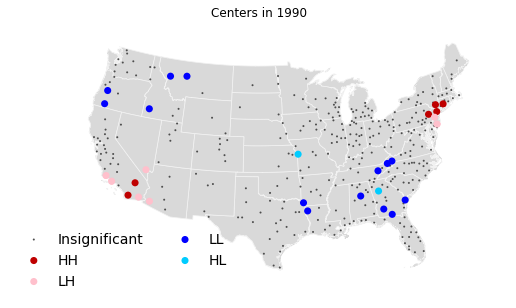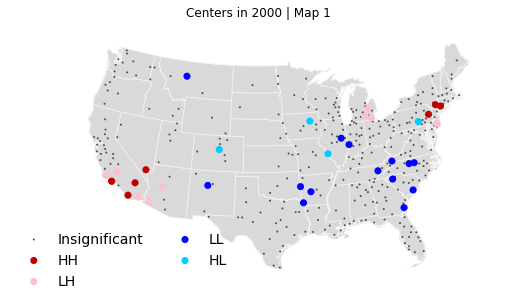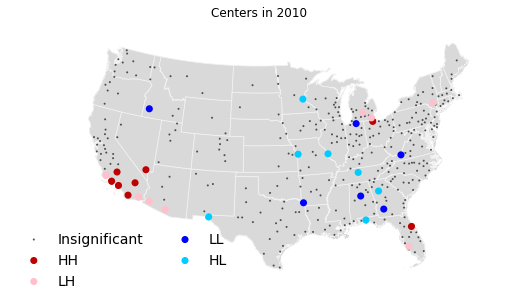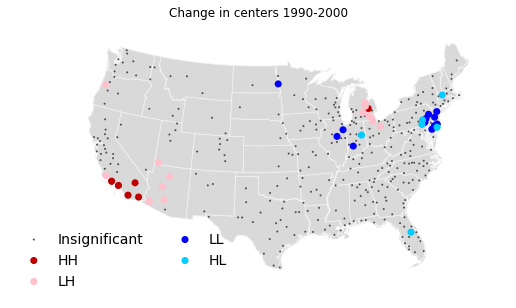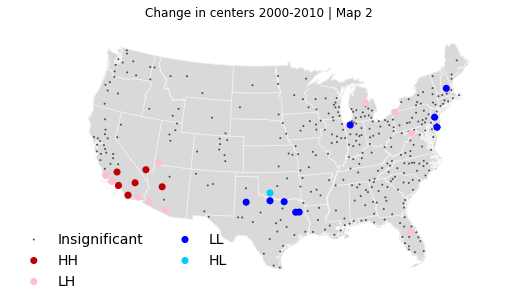## City Characterization¶

Results from the random labeling analysis performed in Section 6 and presented in Tables 5 to 8 of the paper. This basically calculates average values for several variables by type of MSA (no centers, monocentric, polycentric) and tests for statistically significant differences between the averages by using the random labeling technique, borrowed from Rey & Sastré-Gutiérrez (2010). The variables employed are: total population, employment density, income per capita and percentage of people below the poverty line.

Population, income and poverty are also obtained from NHGIS and not redistributed in this context. Employment and density are calculated from the previous data shown in the sections above.

In :
```pop00F='/Users/dani/AAA/LargeData/T-CentersData/attributes/5-pop/pop2000/'
pop00 = pd.concat([tools.load_soc_ec(pop00F+l) for l in os.listdir(pop00F)])
pop00 = pop00.groupby('msa').sum()

sec00F='/Users/dani/AAA/LargeData/T-CentersData/attributes/7-socEc/socEc2000/'
sec00 = pd.concat([tools.load_soc_ec(sec00F+l) for l in os.listdir(sec00F)])
sec00 = sec00.groupby('msa').sum().drop('incpc', axis=1)

msas_soec = db.groupby(['msa', 'year']).apply(\
lambda x: x.groupby('center_id').ngroups)\
.apply(tools._monopoly).unstack()
msas_soec = msas_soec.join(pop00).join(sec00)
msas_soec = msas_soec.join(db[db['year']==2000][['emp', 'Shape_area', 'msa']]\
.groupby('msa').sum())
msas_soec['emp_dens'] = msas_soec['emp'] * 1. / msas_soec['Shape_area']
msas_soec['inc_pc'] = msas_soec['inc'] * 1. / msas_soec['pop']
msas_soec['pct_below'] = msas_soec['below'] * 100. / msas_soec['pop']
msas_soec = msas_soec[[1990, 2000, 2010, \
'pop', 'emp_dens', 'inc_pc', 'pct_below']]
```
In :
```perms = 99
rl90_00 = tools.do_rl(msas_soec, [1990, 2000], perms)
for var in rl90_00:
print "\n\t%s"%var
print rl90_00[var].unstack().fillna('')

print '-------------------------------------------------------------------'
rl_tables = []
rl00_10 = tools.do_rl(msas_soec, [2000, 2010], perms)
for var in rl00_10:
print "\n\t%s"%var
tab = rl00_10[var].unstack().fillna('')
print tab
rl_tables.append(tab)
```
```
pop
2000             empty       monocentric       polycentric
1990
empty        57961.0-*   123688.117647-*           79551.0
monocentric    71914.0  188404.046512-**     416521.833333
polycentric                359553.609756  1749223.21875+**

emp_dens
2000                 empty       monocentric       polycentric
1990
empty        3.97835490525  13.8418248027-**     41.5310305712
monocentric   1.7953228977  23.1552069292-**     32.0401867937
polycentric                    33.7103582425  78.0635013324+**

inc_pc
2000                    empty       monocentric       polycentric
1990
empty           18261.7035593  17865.5425507-**     21530.0851026
monocentric  13672.9440721-**  19173.5165033-**     20090.7586838
polycentric                       19209.1533279  21906.4143172+**

pct_below
2000                 empty      monocentric       polycentric
1990
empty        13.1122651438  14.233278484+**     8.09417857726
monocentric  14.5312456545    12.5869946497     12.4074469974
polycentric                    12.295737511  11.1312509008-**
-------------------------------------------------------------------

pop
2010                 empty       monocentric       polycentric
2000
empty              57961.0           71914.0
monocentric  122379.666667  197135.020833-**     315223.685714
polycentric                    473461.142857  1538691.84956+**

emp_dens
2010                 empty       monocentric      polycentric
2000
empty        3.97835490525      1.7953228977
monocentric  24.2420604204  22.4362925013-**    34.8467702828
polycentric                    28.1182516111  71.709533095+**

inc_pc
2010                 empty       monocentric       polycentric
2000
empty        18261.7035593   13672.9440721-*
monocentric  18383.7643691  18922.0857024-**     20026.9314404
polycentric                    20818.9988839  21555.7755371+**

pct_below
2010                 empty       monocentric       polycentric
2000
empty        13.1122651438     14.5312456545
monocentric  11.0085750881  12.8765604742+**     11.5922490179
polycentric                    12.0246560865  11.3324999094-**

```

These tables can be presented in a more pleasant way, adding the global averages as well the way they are shown in the paper:

In :
```print "Table 5: Size"
print "Global average: %.1f"%msas_soec['pop'].mean()
rl_tables
```
```Table 5: Size
Global average: 640335.4

```
Out:
2010 empty monocentric polycentric
2000
empty 57961.0 71914.0
monocentric 122379.666667 197135.020833-** 315223.685714
polycentric 473461.142857 1538691.84956+**
In :
```print "Table 6: Density"
print "Global average: %.1f"%msas_soec['emp_dens'].mean()
rl_tables
```
```Table 6: Density
Global average: 39.3

```
Out:
2010 empty monocentric polycentric
2000
empty 3.97835490525 1.7953228977
monocentric 24.2420604204 22.4362925013-** 34.8467702828
polycentric 28.1182516111 71.709533095+**
In :
```print "Table 7: Income per capita"
print "Global average: %.1f"%msas_soec['inc_pc'].mean()
rl_tables
```
```Table 7: Income per capita
Global average: 19911.8

```
Out:
2010 empty monocentric polycentric
2000
empty 18261.7035593 13672.9440721-*
monocentric 18383.7643691 18922.0857024-** 20026.9314404
polycentric 20818.9988839 21555.7755371+**
In :
```print "Table 8: Poverty (in %)"
print "Global average: %.1f"%msas_soec['pct_below'].mean()
rl_tables
```
```Table 8: Poverty (in %)
Global average: 12.2

```
Out:
2010 empty monocentric polycentric
2000
empty 13.1122651438 14.5312456545
monocentric 11.0085750881 12.8765604742+** 11.5922490179
polycentric 12.0246560865 11.3324999094-**